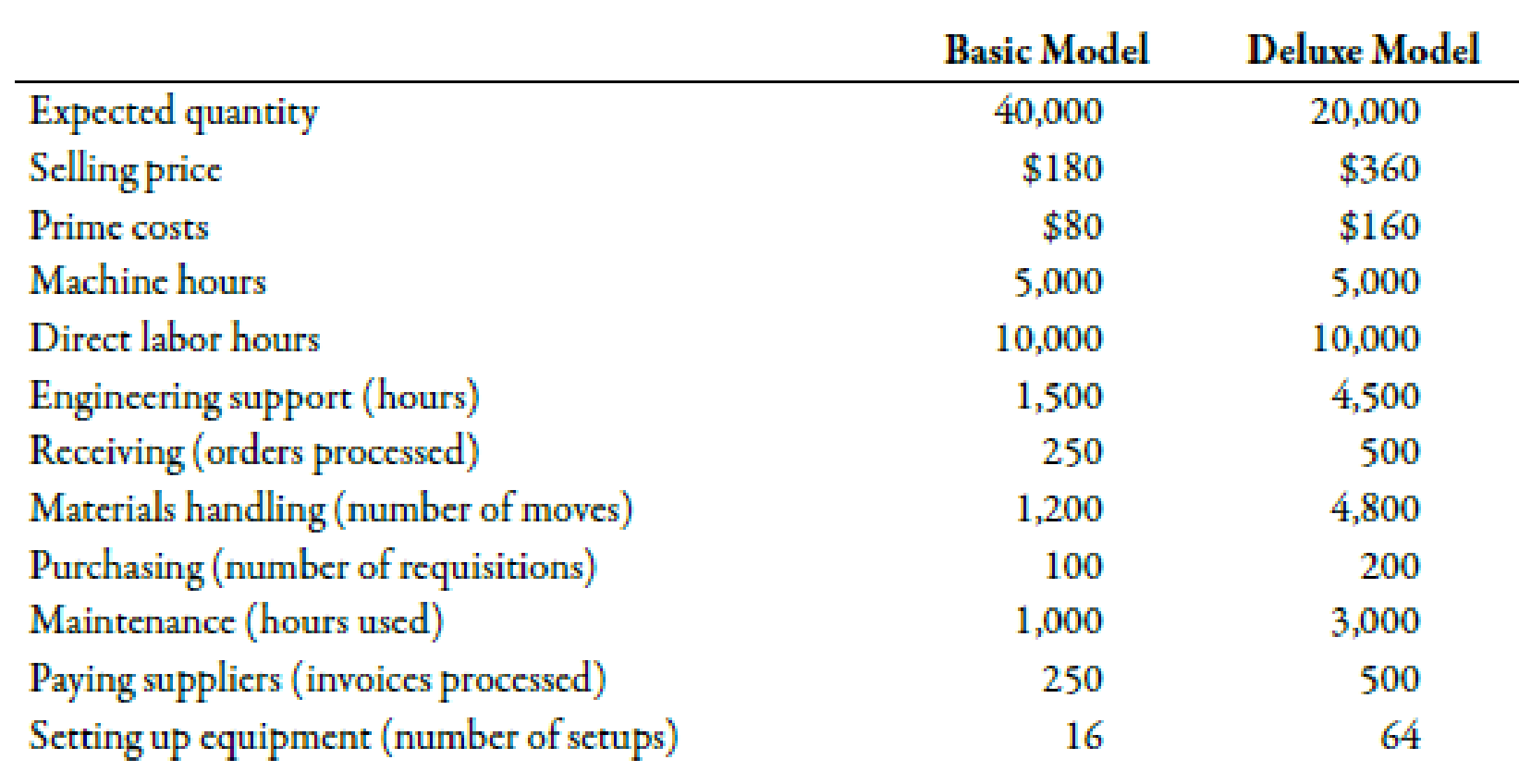Chapter 5, Problem 55P### Managerial Accounting: The Corners...

7th Edition
Maryanne M. Mowen + 2 others
ISBN: 9781337115773

#### Solutions

Chapter
Section### Managerial Accounting: The Corners...

7th Edition
Maryanne M. Mowen + 2 others
ISBN: 9781337115773
Textbook Problem
101 views

# Production-Based Costing versus Activity-Based Costing, Assigning Costs to Activities, Resource DriversWillow Company produces lawnmowers. One of its plants produces two versions of mowers: a basic model and a deluxe model. The deluxe model has a sturdier frame, a higher horsepower engine, a wider blade, and mulching capability. At the beginning of the year, the following data were prepared for this plant:Additionally, the following overhead activity costs are reported:Facility-level costs are allocated in proportion to machine hours (provides a measure of time the facility is used by each product). Receiving and materials handling use three inputs: two forklifts, gasoline to operate the forklift, and three operators. The three operators are paid a salary of $40,000 each. The operators spend 25% of their time on the receiving activity and 75% on moving goods (materials handling). Gasoline costs$3 per move. Depreciation amounts to $8,000 per forklift per year.Required:(Note: Round answers to two decimal places.) 1. Calculate the cost of the materials handling activity. Label the cost assignments as driver tracing or direct tracing. Identify the resource drivers. 2. Calculate the cost per unit for each product by using direct labor hours to assign all overhead costs. 3. Calculate activity rates, and assign costs to each product. Calculate a unit cost for each product, and compare these costs with those calculated in Requirement 2. 4. Calculate consumption ratios for each activity. 5. CONCEPTUAL CONNECTION Explain how the consumption ratios calculated in Requirement 4 can be used to reduce the number of rates. Calculate the rates that would apply under this approach. 1. To determine Compute the costs of the material handling activity and label the activities into driver tracing or direct tracing. Also, identify the resource drivers of the various activities. Explanation Activity Based Costing (ABC): Activity based costing is an apportionment of costs that first considers the activity drivers that helps in the allocation of costs to various activities and then allocates costs to different cost objects by using the drivers.  Particulars Rate (R) ($) Quantity (Q) Amount (R×Q) (\$) Labor 120,000 0

2.

To determine

Compute the costs per unit for each product with the help of direct labor hours.

3.

To determine

Compute the activity rates and assign this cost to each product. Compute unit cost for each product and compare the costs.

4.

To determine

Compute the consumption ratios for each activity.

5.

To determine

Discuss the impact of consumption ratio on reducing the taxes. Also, compute the rate of taxes that would apply under this approach.

### Still sussing out bartleby?

Check out a sample textbook solution.

See a sample solution

#### The Solution to Your Study Problems

Bartleby provides explanations to thousands of textbook problems written by our experts, many with advanced degrees!

Get Started

#### Find more solutions based on key concepts